# A Novel Hybrid MPPT Controller Using (P&O)-neural Networks for Variable Speed Wind Turbine Based on DFIG

A Novel Hybrid MPPT Controller Using (P&O)-neural Networks for Variable Speed Wind Turbine Based on DFIG

Said Azzouz Sabir MessaltiAbdelghani Harrag

Department of Electrical Engineering, Faculty of technology, University of Msila, Msila 28000, Algeria

LGE Laboratory, department of electrical engineering, Faculty of Technology, University of Msila, Msila 28000, Algeria

CCNS Laboratory, Electronics Department, Faculty of Technology, Setif 19000, Algeria

Corresponding Author Email:
sabir.messalti@univ-msila.dz
Page:
23-29
|
DOI:
https://doi.org/10.18280/mmc_a.920104
14 December 2018
|
Accepted:
27 March 2019
|
Published:
31 March 2019
| Citation

OPEN ACCESS

Abstract:

Although the numerous advantages of wind turbine, the nonlinear characteristic (P–Ω) providing a unique maximum power point is the most drawbacks. Therefore a maximum power point tracker is usually adopted, which the Tip-Speed ratio, the perturbation and observation and the optimum torque control methods are widely used. In this article, a novel neural network (ANN) MPPT controller based on perturbation and observation has been projected and studied. The ANN MPPT controller of wind turbine system is established in two independents steps: the offline operation mode is mandatory for training of different neural networks parameters and the Online operation mode where the most advantageous neural network MPPT controller is implanted in wind turbine system. The developed MPPT controller is tested on wind turbine based–DFIG generator, which it is controlled by sliding mode control (SMC). Simulation results are presented and discussed.

Keywords:

neural network MPPT controller, combined MPPT controller, P&O MPPT, DFIG, variable speed wind turbine

1. Introduction

Today, the rapid growth of industry, the huge development of modern lifestyles, the fast consumption of the fossil fuel reserves and the pollution caused by the traditional energy sources have encouraged many countries and governments to develop a new efficient energy sources. The new energy strategy uses new forms of green energy well-known "renewable energy" to address the global challenges of clean energy and able to ensure a part of energy production. These energies include the solar, rain, wind; tides, geothermal heat and various forms of biomass are renewable and can be physically replenished continuously. Wind energy is an attractive source of energy and it represent a great potential for power production and consequently it has been developed and implemented in many countries due to its advantages of clean, safe energy resource and resource abundance [1-5].

Although the numerous advantages of wind turbine, many drawbacks can be noted such as the random nature of wind speed leading to the fluctuation of the produced wind turbine power. In addition, the wind turbine has a nonlinear characteristic (P–Ω) which the maximum power point (MPP) is guaranteed in only one point, depending on wind speed and speed ratio. Therefore, the wind turbine system must be calculated to drive the system at their maximum output power for any conditions. Therefore, many MPPT methods have been proposed and implemented [6-16]. In which, The developed MPPT controllers can be classified in two groups: i) the first one is the conventional methods , it includes optimum torque control (OTC) , tip speed ratio (TSR) , perturbation and observation (P&O) [4-10], and power speed feedback (PSF) , and ii) the second group or intelligent methods  uses fuzzy logic, neural-network [11-15], and particle swarm optimization .

In recent years, neural network technique has demonstrated new solution in wind turbine systems. Due to it can be trained off-line, and then used in the on-line physical application . The major benefit of neural network is that it does not need an exact mathematical model and be capable of identify compound nonlinear relationships between dependent and independent variables.

In this document, a new ANN MPPT controller using the famous perturbation and observation (P&O) has been projected and looked for. The necessary ANN model training data are calculated using the classical (P&O) rules [17-18]. The proposed MPPT controller is tested on wind turbine controlled by sliding mode control (SMC). Simulation results are presented and discussed.

2. Modeling of Wind Turbine and DFIG

2.1 Modeling of wind turbine

The mechanical power of wind turbine is written by [3, 10]:

$P_{aer}=\frac{1}{2} C_{p}(\lambda, \beta) \rho S v_{w i n d}^{3}$     (1)

where Paer: is the extracted power from the wind turbine;

$\lambda=\frac{R \Omega_{t}}{V_{\text {wind}}}$     (2)

The wind turbine efficiency Cp is given :

$C_{p}(\lambda, \beta)=(0.35-0.0167(\beta-2)) \sin \left(\frac{\pi(\lambda+0.1)}{14.34-0.3(\beta-2)}\right)-(0.00184(\lambda-3)(\beta-2))$                         (3)

Based on the previous equations, a functional block diagram model developed under Simulik of wind turbine system is presented in Figure 1.

## 1-shang_.png## 1-xia_.png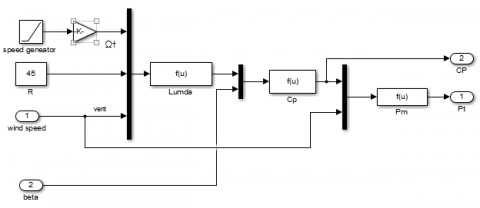Figure 1. Bloc Simulink of wind turbine

## 2.pngFigure 2. Power coefficients Cp(λ) of 3MW wind turbine 

## 3.pngFigure 3. Power versus rotational speed of generator for turbine 3 MW 

The characteristics of the typical captured output power for different pitch angles b (2, 4 to 14) are showed in Figure 2. It can be observed that captured output power of wind turbine depends on pitch angles and it is clear that there is one speciﬁc point (CPmax-lopt) at which the turbine is maximized. Hence, for each wind speed, there is a one turbine speed that provides a maximum output power as shown in Figure 3.

2.2 DFIG model and control

Today, doubly fed induction generators become the most popular variable speed wind turbine concept [20-22]. These class of generators are commonly selected as one of the appropriate wind energy conversion systems since it is able to operate in wide range of speed variation approximately the synchronous speed ±30 %, the independent active and reactive control possibilities and accessibility to the stator and rotor providing the opportunity to control active and reactive powers as well as power factor. The Wind turbine with DFIG system is exposed in Figure 4.

## 4.png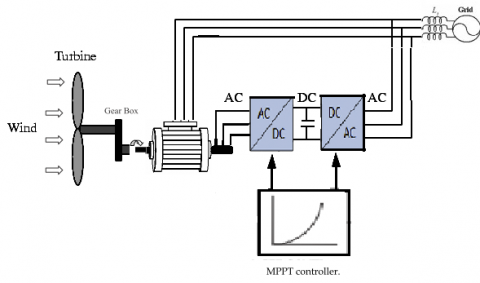Figure 4. The Wind turbine based on DFIG

The DFIG model is expressed as follows [20-22]:

$\left\{\begin{array}{l}{V_{d s}=-R_{s} \cdot I_{d s}+\frac{d}{d t} \phi_{d s}-\omega_{s} \phi_{q s}} \\ {V_{q s}=-R_{s} \cdot I_{q s}+\frac{d}{d t} \phi_{q s}+\omega_{s} \phi_{d s}} \\ {V_{d r}=R_{r} I_{d r}+\frac{d}{d t} \phi_{d r}-\omega_{r} \phi_{q r}} \\ {V_{q r}=R_{r} I_{q r}+\frac{d}{d t} \phi_{q r}+\omega_{r} \phi_{d r}}\end{array}\right.$     (4)

$\left\{\begin{array}{l}{\phi_{d s}=-L_{s} I_{d s}+M \cdot I_{d r}} \\ {\phi_{q s}=-L_{s} \cdot I_{q s}+M \cdot I_{q r}} \\ {\phi_{d r}=L_{r} \cdot I_{d r}-M I_{d s}} \\ {\phi_{q r}=L_{r} \cdot I_{q r}-M \cdot I_{q s}}\end{array}\right.$     (5)

The DFIG torque is expressed by:

$C_{e m}=p \frac{M}{L_{s}}\left[\varphi_{q s} i_{d r}-\varphi_{d s} i_{q r}\right]$     (6)

To accomplish independent control [19-24], the subsequent equations can be obtained:

$\phi_{d s} = \phi_{s}$ and $\phi_{q s} = 0$     (7)

The voltage Vdr and Vqr shown in Figure 5 are expressed respectively by:

$\left\{\begin{array}{l}{V_{d r}=R_{r} I_{d r}-\mathrm{g} \omega_{s}\left(L_{r}-\frac{M^{2}}{L_{s}}\right) I_{q r}} \\ {V_{q r}=R_{r} I_{q r}+\mathrm{g} \omega_{s}\left(L_{r}-\frac{M^{2}}{L_{s}}\right) I_{d r}+\mathrm{g} \omega_{s} \frac{M \phi_{s}}{L_{s}}}\end{array}\right.$     (8)

## 5.png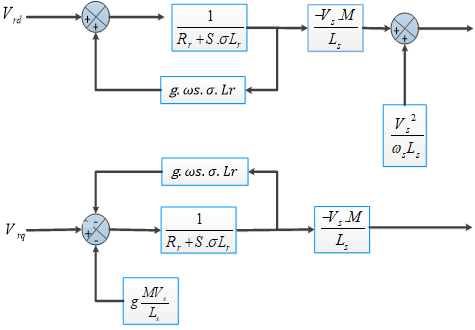Figure 5. Field oriented control of DFIG

2.3 Sliding mode control of DFIG

Recently, the sliding mode control (SMC) has been considered as one of most used techniques for DFIG drive control among nonlinear control strategies, due to its simplicity, easy implementation and robustness [25-27]. The sliding mode control is based on three steps: the first is the choice of control surface, the second is convergence condition, and the third is control law design.

SMC algorithm consists to calculate the equivalent and discontinuous components of control variable from an adequate surface of sliding mode chosen. In this case we chose the error as being the sliding surface.

The control algorithm is defined by following expression:

$u=u^{e q}+u^{n}$     (9)

where

u is the control vector, $u^{e q}$ is the equivalent control vector,

$u^{n}$ is the switching part of the control (the correction factor)

$u^{e q}$ can be obtained by considering the condition for the sliding regime, = 0

The control law is defined as follows:

$\left\{\begin{array}{lll}{u^{n}=u^{\max (s(X) / \theta)}} & {} \\ {\operatorname{sat}(s(X) / \theta)\left\{\begin{array}{ll}{\operatorname{sign}(s)} & {\text {if }} & {|s|>\theta} \\ {s / \theta} & {\text { if }} & {|s|<\theta}\end{array}\right.}\end{array}\right.$     (10)

The sliding surface is calculated by the general equation J.J. Slotine:

$s(X)=\left(\frac{d}{d t}+\lambda\right)^{n-1} e$     (11)

where the convergence condition is defined by the equation Lyapunov $s(x) \dot{s}(x)<0$.

2.3.1 Active power control

For n=1, the sliding surface representing the error between the measured and reference active power is given by:

$s(P)=\left(P_{s-r e f}-P_{s}\right)$     (12)

The derivative of the surface is given by

$\dot{s}(P)=\left(\dot{P}_{s-r e f}-\dot{P}_{s}\right)$     (13)

By replacing active power equation in the equation of the switching surface, the expression of the surface becomes:

$\dot{s}(P)=\left(\dot{P}_{s-r e f}+\frac{V_{s} M}{L_{s}} \dot{I}_{r q}\right)$     (14)

From the equation (11) (During the sliding mode and in permanent regime), we can write

$\dot{I}_{r q}=\frac{1}{L_{r} \sigma} \cdot\left(V_{r q}-R_{r} I_{r q}\right)$     (15)

The control law design Vrq is given by:

$V_{r q}=V_{r q}^{e q}+V_{r q}^{n}$     (16)

Replacing equation (16) in equation (14), the equation we have

$\dot{s}(P)=\left(\dot{P}_{s-r e f}+\frac{V_{s} M}{L_{s} L_{r} \sigma}\left(\left(V_{r q}^{e q}+V_{r q}^{n}\right)-R_{r} I_{r q}\right)\right)$     (17)

During the sliding mode and in permanent regime, we have:

$s(P)=0, \dot{s}(P)=0, \quad V_{r q}^{n}=0$

Replacing in previous equation, the equivalent control $V_{r q}^{e q}$ and correction factor are given by:

$\left\{\begin{array}{l}{V_{r q}^{e q}=\dot{P}_{s-r e f} \frac{L_{s} L_{r} \sigma}{V_{s} M}+R_{r} I_{r q}} \\ {V_{r q}^{n}=K_{V r q} \operatorname{sign}(S(P))}\end{array}\right\}$          (18)

where: $K_{V r q}$ positive constant .

2.3.2 Reactive power control

For n=1, the reactive power control surface becomes is given by

$s(\Phi)=\left(\Phi_{s-r e f}-\Phi_{s}\right)$     (19)

The derivative of the surface is given by

$\dot{s}(\Phi)=\left(\dot{\Phi}_{s-r e f}-\dot{\Phi}_{s}\right)$     (20)

From the equation (11) the current expression is given by

$\dot{I}_{r d}=\frac{1}{L_{r} \sigma}\left(V_{r d}-R_{r} I_{r d}\right)$     (21)

The control law design Vrd is given by:

$V_{r d}=V_{r d}^{e q}+V_{r d}^{n}$     (22)

From equation (24), (21) and (22), the derivative of the surface can be expressed by:

$\dot{s}(\Phi)=\left(\dot{\Phi}_{s-r e f}+\frac{V_{s} M}{L_{s} L_{r} \sigma}\left(\left(V_{r d}^{e q}+V_{r d}^{n}\right)-R_{r} I_{r d}\right)\right)$     (23)

During the sliding mode and in steady state

$s(\Phi)=0, \dot{s}(\Phi)=0, \quad V_{r d}^{n}=0$

Therefore, the equivalent control $V_{r d}^{e q}$ and the switching term is given by can be expressed by:

$\left\{\begin{array}{l}{V_{r d}^{e q}=-\dot{\Phi}_{s-r e f} \frac{L_{s} L_{r} \sigma}{V_{s} M}+R_{r} I_{r d}} \\ {V_{r d}^{n}=K_{V r d} \operatorname{sign}(S(\Phi))}\end{array}\right\}$          (24)

where: $K_{V r d}$  is positive constant .

## 6-shang_.png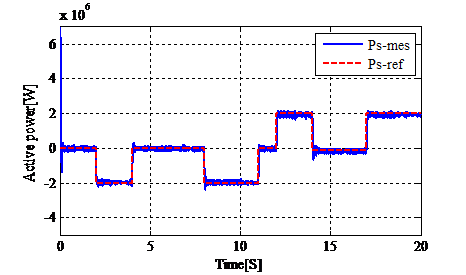## 6-xia_.pngFigure 6. Active and reactive power of the DFIG using S.M.C

The obtained simulation results of the DFIG using S.M.C is shown in Figure 6, in which the produced active and reactive power follow perfectly their references.

3. Proposed ANN MPPT Controller Based on P&O Method

3.1 Perturb & observe (P&0) method

The perturb & observe (P&O) MPPT controller is widely applied, it can be used by small step size disturbing the control variable and analyzing the resulting raising or decreasing in generated power (the controller must move it right or left of the MPP) as shown in Figure 7 presenting the output power of 3MW wind turbine since a function of generator speed for its nominal wind speed (13 m/s). If it results in the increase in power, then the similar perturbation is useful for the next control occurrence. Otherwise the sign of the perturbation is inverted to track in the way of increasing power. Once the stable state is achieved the process oscillates about the MPP.

## 7.png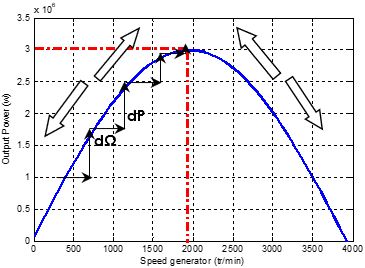Figure 7. Maximal power curve P(Ω) of 3MW wind turbine

## 8.png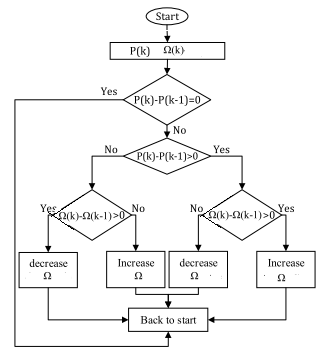Figure 8. Diagram of the P&O method 

Figure 8 explains the ﬂowchart of the P&O strategy. The output power is maximum at a rotor speed well known most favorable rotor speed Ωopt, corresponding to optimum tip speed ratio lopt. So, to produce maximum possible power, the turbine must be driven at Ωopt. This is achievable by scheming the rotational speed of the turbine so that it always turns at the finest speed rotation.

3.2 Proposed ANN MPPT

Technological developments in recent years has allowed scientists to develop and refine methods for different applications, especially in the renewable energies areas, which they require the use of chic techniques for an perfect estimation of the accessible energy prospective and any successful control of systems operation .

Over the last few decades, many control strategies have been developped. Artificial neural networks techniques have been selected as one of  the best solution  for  computational system since they offer many benefits compared to the conventional computational systems [8, 17-18].

The proposed artificial neural network (ANN) MPPT controller is established on the similar law of Perturbation and Observation (P&O) method decreasing or increasing the generator speed regarding the sign of (dP/dΩ), the fundamental rule of ANN MPPT controller is recapitulated in Table 1.

Table 1. Fundamental rule of artificial neural network MPPT controller

 dP dΩ dP/ dΩ Increase /deacrease of Ω + + + Ω(s) = Ω(s-1) + ∆Ω + - - Ω(s) = Ω(s-1) - ∆Ω Ω - + - Ω(s) = Ω(s-1) - ∆Ω Ω - - + Ω(s) = Ω(s-1) + ∆Ω Ω

The developed ANN system operates in two epochs:

1) The offline epoch necessary to trying different set of neural network parameters to find the most favorable neural network controller (activation function, organization, and training algorithm), when the training and testing performed in off-line, a neural network bloc model is obtained, and then it is introduced on-line in MPPT .

2) The online epoch that employs the best ANN MPPT regulator to track the MPP point. The fundamental diagram of wind turbine system is demonstrated in Figure 9.

Figure 10 shows the ANN MPPT regulator developped via Simulink.

## 9.png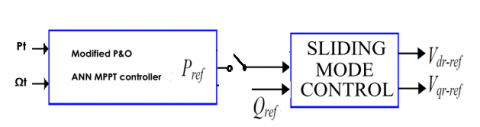Figure 9. Block diagram of the wind turbine system control

## 10.png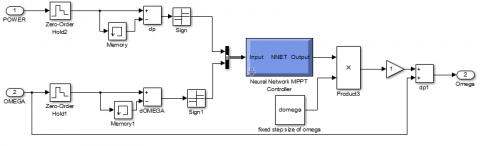Figure 10. ANN MPPT regulator based on P&O rule

## 11.png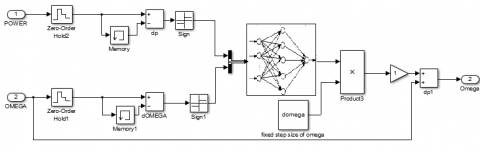Figure 11. Developed ANN MPPT regulator using P&O rule

A simulation study was tested for the wind speed estimation and tracking control of the most favorable maximum power using a feed-forward neural networks having three layers. The inputs variables of the projected ANN MPPT controller are: the output power (P) and generator speed (dΩ). However, the output ANN parameter is the consequent rising or lowering of generator speed +/_∆Ω.

4. Simulation and Results

In this manuscript segment, the competence of the projected artificial neural network MPPT regulator using P&O rule is presented. A wind energy conversion system based on 3MW wind turbine connected to DFIG is employed.

The simulation results have been establised using Simulink environment using the wind speed proﬁle depicted in Figure 12.

Figures 13, 14 and 15 show the power coefficient, the output power and the optimal turbine rotational speeds, respectively, determined based on the optimum operating points of the system.

From figures, its clear that the wind energy- DFIG system operates at its maximum power which it can be verified for nominal wind speed (13m/s). The power coefficient and output power correspond exactly at it nominal value 0.35 and 3MW, respectively.

## 12.png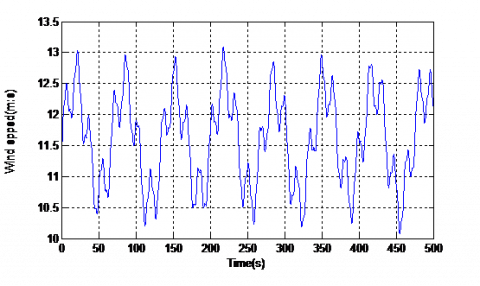Figure 12. Wind speed proﬁle

## 13.png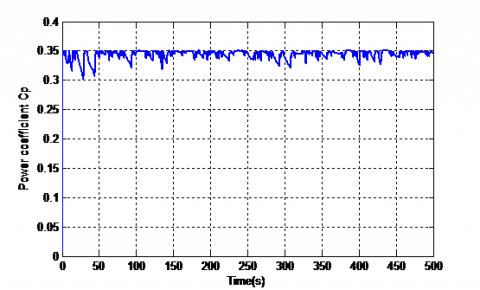Figure 13. Power coefficient Cp

## 14.pngFigure 14. Tracking MPPT power ANN-P&O

## 15.png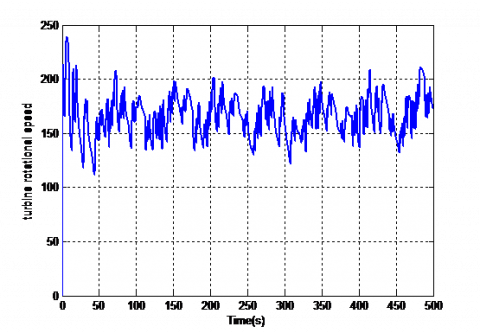Figure 15. Turbine rotational speed

5. Conclusion

In this article, a novel ANN MPPT regulator using (P&O) rules has been presented considering changeable speed wind turbine moving doubly-fed induction generator (DFIG). Modeling and control strategies of the generally system have been developed. Simulation results have carried out using Simulink under randomly and fast changeable wind rapidity. Obtained results exhibit the elevated performance of proposed ANN MPPT regulator. The simulation results are close to the theoretically expected results. The advantages of this proposed method include: good accuracy, does not require information of power coefficient Cpmax and the tip speed ratio lopt and the characteristic curve of wind turbine. In addition, a variable step size can be used in the proposed neural Network MPPT controller for achieving better performances. This last feature will be considered for our future work.

References

 Suresh, K., Babu, A.R.V., Venkatesh, P.M. (2018). Experimental investigations on grid integrated wind energy storage system using neuro fuzzy controller. Modelling, Measurement and Control A, 91(3): 123-130. https://doi.org/10.18280/mmc_a.910304

 Suresh, K., Babu, A.R.V., Venkatesh, P.M. (2018). Experimental investigations on grid integrated wind energy storage system using neuro fuzzy controller. Modelling, Measurement and Control A, 91(3): 123-130.

 Magrini, A., Lazzari, S., Marenco, L., Guazzi, G. (2018). Cost optimal analysis of energy refurbishment actions depending on the local climate and its variations. Mathematical Modelling of Engineering Problems, 5(3): 268-274. https://doi.org/10.18280/mmep.050321

 Manukonda, D., Gorantla, S.R. (2018). A fuzzy logic controller based vortex wind turbine for commercial applications. Modelling, Measurement and Control A, 91(2): 54-58. https://doi.org/10.18280/mmc_a.910204

 Messaoud, M., Abdessamed, R. (2011). Modeling and optimization of wind turbine driving permanent magnet synchronous generator. Jordan Journal of Mechanical and Industrial Engineering, 5(6): 489-494.

 Tanaka, T., Toumiya, T., Suzuki, T. (1997). Output control by hill-climbing method for a small scale wind power generating system. Renewable Energy, 12(4): 387-400. https://doi.org/10.1016/S0960-1481(97)00055-4.

 Hosseini, S.E., Abdul Wahid, M. (2014). The role of renewable and sustainable energy in the energy mix of Malaysia: A review. International Journal of Energy Research, 38(14): 1769-1792. https://doi.org/10.1002/er.3190

 Musunuri, S., Ginn, H.L. (2011). Comprehensive review of wind energy maximum power extraction algorithms. In 2011 IEEE power and energy society general meeting, pp. 1-8. https://doi.org/10.1109/PES.2011.6039023

 Saad, N.H., El-Sattar, A.A., Metally, M.E. (2018). Artificial neural controller for torque ripple control and maximum power extraction for wind system driven by switched reluctance generator. Ain Shams Engineering Journal, 9(4): 2255-2264. https://doi.org/10.1016/j.asej.2017.03.005

 Mahvash, H., Taher, S.A., Rahimi, M. (2017). A new approach for power quality improvement of DFIG based wind farms connected to weak utility grid. Ain Shams Engineering Journal, 8(3): 415-430. https://doi.org/10.1016/j.asej.2015.09.001

 Goh, H.H., Anwar, M.S., Chua, Q.S., Ling, C.W., Goh, K.C. (2017). Analysis between perturb & observe controller and fuzzy logic controller for a photovoltaic system with CUK and SEPIC converter. Journal of Telecommunication, Electronic and Computer Engineering (JTEC), 9(3-7): 81-88.

 Wang, Q., Chang, L. (2004). An intelligent maximum power extraction algorithm for inverter-based variable speed wind turbine systems. IEEE TRANSACTIONS on Power Electronics, 19(5): 1242-1249. https://doi.org/10.1109/TPEL.2004.833459

 Algazar, M.M., El-Halim, H.A., Salem, M.E.E.K. (2012). Maximum power point tracking using fuzzy logic control. International Journal of Electrical Power & Energy Systems, 39(1): 21-28. https://doi.org/10.1016/j.ijepes.2011.12.006

 Mohamed, A.Z., Eskander, M.N., Ghali, F.A. (2001). Fuzzy logic control based maximum power tracking of a wind energy system. Renewable Energy, 23(2): 235-245. https://doi.org/10.1016/S0960-1481(00)00099-9

 Jabr, H.M., Lu, D., Kar, N.C. (2011). Design and implementation of neuro-fuzzy vector control for wind-driven doubly-fed induction generator. IEEE Transactions on Sustainable Energy, 2(4): 404-413. https://doi.org/10.1109/TSTE.2011.2160374

 Pucci, M., Cirrincione, M. (2010). Neural MPPT control of wind generators with induction machines without speed sensors. IEEE Transactions on Industrial Electronics, 58(1): 37-47. https://doi.org/10.1109/TIE.2010.2043043

 Tianpei, Z., Wei, S. (2013). MPPT method of wind power based on improved particle swarm optimization. TELKOMNIKA Indonesian Journal of Electrical Engineering, 11(6): 3206-3212.

 Messalti, S., Boudjellal, B., Said, A. (2015). Artificial neural networks controller for power system voltage improvement. In IREC2015 The Sixth International Renewable Energy Congress, pp. 1-6. https://doi.org/10.1109/IREC.2015.7110897

 Messalti, S., Harrag, A., Loukriz, A. (2017). A new variable step size neural networks MPPT controller: Review, simulation and hardware implementation. Renewable and Sustainable Energy Reviews, 68(1): 221-233

 Gaillard, A. (2010). Système éolien basé sur une MADA: Contribution à l'étude de la qualité de l'énergie électrique et de la continuité de service. Doctoral dissertation, Université Henri Poincaré-Nancy 1.

 Zemmit, A., Messalti, S., Harrag, A. (2017). A new improved DTC of doubly fed induction machine using GA-based PI controller. Ain Shams Engineering Journal, 9(4): 1877-1885. https://doi.org/10.1016/j.asej.2016.10.011

 Akhmatov, V. (2003). Variable-speed wind turbines with doubly-fed induction generators Part IV: Uninterrupted operation features at grid faults with converter control coordination. Wind Engineering, 27(6): 519-529. https://doi.org/10.1260/030952403773617481

 Muller, S., Deicke, M., De Doncker, R.W. (2002). Doubly fed induction generator systems for wind turbines. IEEE Industry Applications Magazine, 8(3): 26-33. https://doi.org/10.1109/2943.999610

 Yamamoto, M., Motoyoshi, O. (1991). Active and reactive power control for doubly-fed wound rotor induction generator. IEEE Transactions on Power Electronics, 6(4): 624-629. https://doi.org/10.1109/63.97761

 Adjoudj, M., Abid, M., Aissaoui, A.G., Ramdani, Y., Bounoua, H. (2011). Sliding mode control of a doubly fed induction generator for wind turbines. Rev. Roum. Sci. Techn.–Électrotechn. etÉnerg, 56(1): 15-24.

 Boukezzar, B., M'Saad, M. (2008, June). Robust sliding mode control of a DFIG variable speed wind turbine for power production optimization. In 2008 16th Mediterranean Conference on Control and Automation, pp. 795-800. https://doi.org/10.1109/MED.2008.4602145

 Liu, X., Han, Y. (2014). Sliding mode control for DFIG-based wind energy conversion optimization with switching gain adjustment. In Proceeding of the 11th World Congress on Intelligent Control and Automation, pp. 1213-1218. https://doi.org/10.1109/WCICA.2014.7052892